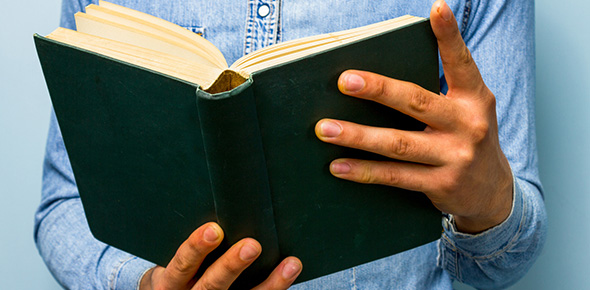20 Questions | Total Attempts: 251SettingsThis quiz covers the reading assignment from Chapter 2. 3-4 on the topics of molecules, types of bonds, and chemical reactions.

• 1.
Please explain the type of bonding in Fig. 2.16 of your textbook in as much detail as possible (describe WHAT is happening, WHY it is happening, WHEN this is most likely to occur -- USE vocabulary words whenever possible!)
• 2.
Why does a greater concentration of reactants INCREASE the rate of a reaction?
• 3.
Which of these relationships is true of an uncharged atom?
• A.

Atomic mass is equal to the number of electrons.

• B.

Number of protons is equal to the number of electrons.

• C.

Number of neutrons is equal to the number of protons.

• D.

Atomic mass is equal to the atomic number.

• E.

Number of electrons is equal to the number of neutrons.

• 4.
Which of the following determines the types of chemical reactions that an atom participates in?
• A.

Its atomic mass

• B.

The number of electrons in the innermost electron shell

• C.

The number of electrons in the outermost electron shell

• D.

The number of protons it contains

• E.

Its atomic number

• 5.
In figure 2.14 of your textbook a Sodium (Na) atom is transferring one of its electrons to an atom of Chlorine (Cl).  In the end both are satisfied with an outer electron shell containing 8 electrons - Na with a charge of +1 and Cl with a charge of -1.  This is an example of ________________ bonding.
• 6.
A _________________ is two or more atoms held together by covalent bonds.
• 7.
A ____________________ is a molecule made of more than 2 or more elements.
• 8.
When Carbon and Hydrogen atoms meet, what type of a bond do they make?
• A.

Hydrogen

• B.

Nonpolar covalent

• C.

Ionic

• D.

Polar covalent

• 9.
Which of the following represent the 2 weakest types of bonding?
• A.

Ionic

• B.

Hydrogen

• C.

Covalent

• D.

Van der Waals

• 10.
The Greek "delta" symbol indicates a ________________ positive or negative charge on an atom.
• 11.
__________________________ Van der Waals interactions are very weak, but many of them combined, as in the gecko example in your textbook, can be extremely powerful.
• 12.
The shape of a molecule determines:
• A.

Its ability to bond with other biological molecules

• B.

Its ability to be recognized by other biological molecules

• C.

Its ability to form weak interactions with and temporarily interact with other biological molecule

• D.

Its ability to mimic other biological molecules and their functions

• 13.
Elements or molecules listed to the LEFT of a reaction arrow are called the _________________________.
• 14.
Molecules or elements listed to the RIGHT of a reaction arrow are called the __________________________.
• 15.
_______________________ tell you how many of each molecule are present and reacting in a chemical equation.
• 16.
In Fig. 2.19 of your textbook a sprig of Elodea is shown as an example of photosynthesis.  The bubbles being produced are made of ___________________.
• 17.
Look at the balanced chemical equation for the creation of water on page 42 in your textbook.  According to this reaction _____ molecule(s) of water is/are being produced.
• 18.
Please check ALL of the following that represent possible %s of reactants and products at the dynamic equilibrium of a reaction.
• A.

10%/90%

• B.

30%/70%

• C.

10%/30%

• D.

50%/35%

• E.

80%/20%

• 19.
All chemical reactions are reversible.
• A.

True

• B.

False

• 20.
At a chemical reaction's dynamic equilibrium there are always equal amounts of reactants and products.
• A.

True

• B.

False

Related TopicsBack to top